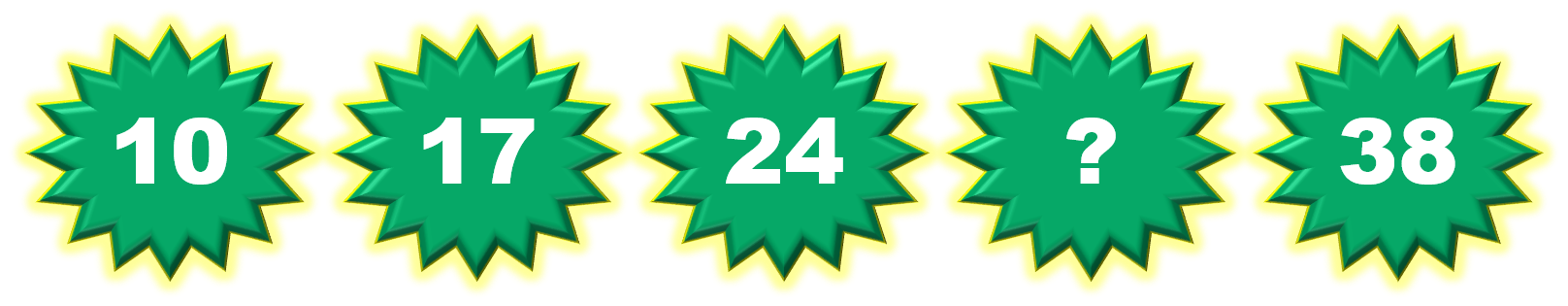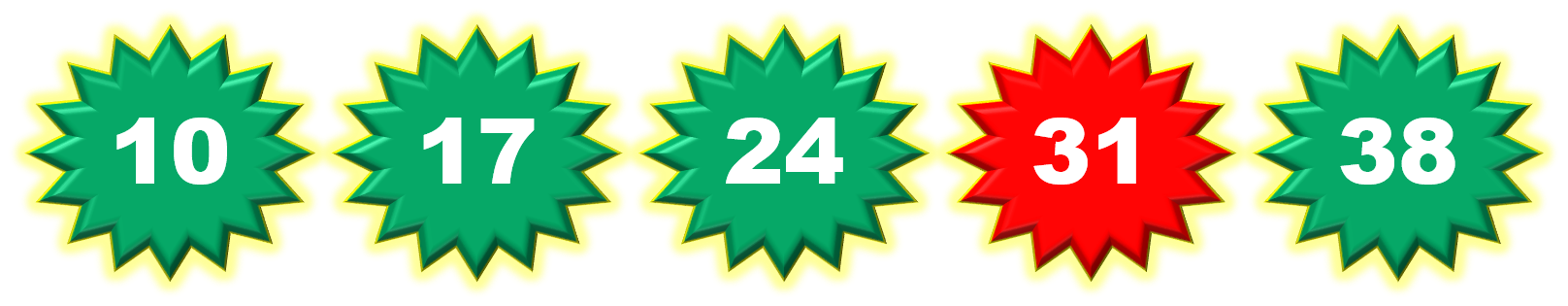1
visibility

Consider the given number pattern and identify the missing number.• A

34

• B

33

• C

32

• D

31

The correct answer is 31.

Solution:

Considering the given number pattern.Here, the numbers are increasing.
So, to find the missing number, we first find the rule.

Note that:
10+ 7 = 17
17 + 7 = 24
24 + 7 = 31
31 + 7 = 38

So, the rule is "add 7".Then, the missing number is 31.